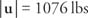## The Rectangular Coordinate System

The following discussion is limited to vectors in a two‐dimensional coordinate plane, although the concepts can be extended to higher dimensions.

If vector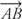is shifted so that its initial point is at the origin of the rectangular coordinate plane, it is said to be in standard position. If vectoris equal to vectorand has its initial point at the origin, it is said to be the standard vector for. Other names for the standard vector include radius vector and position vector (Figure  1).Figure 1
Vectors drawn on a plane.

Vectoris the standard vector for all vectors in the plane with the same direction and magnitude as. In order to find the standard vector for a geometric vector in the coordinate plane, only the coordinates of point P must be found because point 0 is at the origin. If the coordinates of point A are ( x a, y a ) and the coordinates of point B are ( x b, y b), then the coordinates of point P are ( x bx a, y ab − y a).

Example 1: If the endpoints of a vectorhave coordinates of A(−2, −7) and B (3, 2), then what are the coordinates of point P such thatis a standard vector and=(see Figure  2)?Figure 2
Drawing for Example 1.

If the coordinates of point P are ( x, y),An algebraic vector is an ordered pair of real numbers. An algebraic vector that corresponds to standard geometric vectoris denoted as ⟨ a, b⟩ if terminal point P has coordinates of (a, b). The numbers a and b are called the components of vector ⟨ a, b⟩ (see Figure 3 ).Figure 3
Components of a vector.

If a, b, c, and d are all real numbers such that a = c and b = d, then vector v = ⟨ a, b⟩ and vector u = ⟨ c, d⟩ are said to be equal. That is, algebraic vectors with equal corresponding components are equal. If both components of a vector are equal to zero, the vector is said to be the zero vector. The magnitude of a vector v = ⟨a, b⟩ is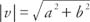.

Example 2: What is the magnitude of vector u = ⟨3, −5⟩?Vector addition is defined as adding corresponding components of vectors—that is, if v = ⟨ a, b⟩ and u = ⟨c, d⟩, then v + u = ⟨a + c, b + d⟩ (Figure 4 ).Figure 4

Scalar multiplication is defined as multiplying each component by a constant—that is, if v = ⟨a, b⟩ and q is a constant, then q v = q⟨a, b⟩ = ⟨qa, qb⟩.

Example 3: If v = ⟨8, −2⟩ and w = ⟨3, 7⟩ then find 5 v −2 w.A unit vector is a vector whose magnitude is 1. A unit vector v with the same direction as a nonzero vector u can be found as follows:Example 4: Find a unit vector v with the same direction as the vector u given that u = ⟨7, − 1⟩.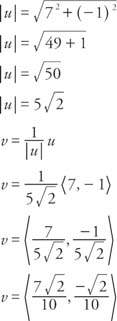Two special unit vectors, i = ⟨1, 0⟩ and j = ⟨0, 1⟩, can be used to express any vector v = ⟨a, b⟩.Example 5: Write u = ⟨5, 3⟩ in terms of the i and j unit vectors (Figure ).Figure 5
Drawing for Example 5.Vectors exhibit algebraic properties similar to those of real numbers (Table  1).Example 6: Find 4 u + 5 v if u = 7 i − 3 j and v = −2 i + 5 j.Given two vectors, u = ⟨ a, b⟩ = a i + b j and v = ⟨c, d⟩ = c i + d j, the dot product, written as u· v, is the scalar quantity u ˙ v = ac + bd. If u, v, and w are vectors and q is a real number, then dot products exhibit the following properties:The last property, u ˙ v = | u| | v| cos α, can be used to find the angle between the two nonzero vectors u and v. If two vectors are perpendicular to each other and form a 90° angle, they are said to be orthogonal. Because cos 90° = 0, the dot product of any two orthogonal vectors is 0.

Example 7: Given that u = ⟨ 5, −3⟩ and v = ⟨6, 10⟩, show that u and v are orthogonal by demonstrating that the dot product of u and v is equal to zero.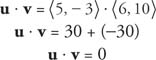Example 8: What is the angle between u = ⟨5, −2⟩ and v = ⟨6, 11⟩?An object is said to be in a state of static equilibrium if all the force vectors acting on the object add up to zero.

Example 9: A tightrope walker weighing 150 pounds is standing closer to one end of the rope than the other. The shorter length of rope deflects 5° from the horizontal. The longer length of rope deflects 3°. What is the tension on each part of the rope?

Draw a force diagram with all three force vectors in standard position (Figure  6).Figure 6
Drawing for Example 9.The sum of the force vectors must be zero for each component.

For the i component: − | u|cos 5° + | v| cos 3° = 0

For the j component: | u| sin5° + |v| cos 3° − 150 =

Solve these two equations for | u| and | v|: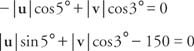Substituting the values for the sines and cosines: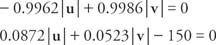Multiply the first equation by 0.0872 and the second by 0.9962:Add the two equations and solve for | v|: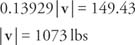Substitute and solve for | u|: